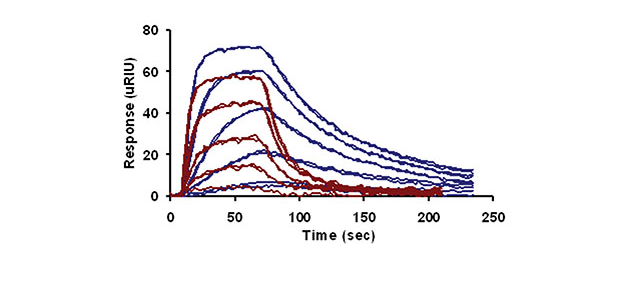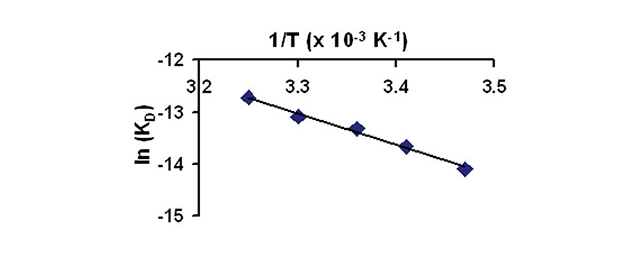XanTec bioanalytics GmbH

# Products | SPR Biosensors | Application note 03: Thermodynamic Analysis

## Thermodynamic Analysis

### Thermodynamic Investigation of an Enzyme-Inhibitor Pair

Understanding the thermodynamics of a system gives valuable insight into the mechanism and forces leading to an interaction. The SR7000DC system provides the opportunity to acquire thermodynamic data for molecular interactions of interest owing to its precise temperature control from 10 oC below ambient to 90 oC. This Application note utilizes the SR7000DC system to characterize the thermodynamics of a small molecule inhibitorenzyme pair, specifically carbonic anhydrase II (CAII) and 4-carboxybenzenesulfonamide (4-CBS). The binding constants of this interaction are measured with respect to temperature to thermodynamically profile this interaction.

#### Experimental

The experimental conditions for this assay are summarized below:

• Ligand: CAII
• Analyte: 4-CBS
• Analyte Concentrations: 20,6.7,2.2,0.74,0.25,0.082 μM
• Temperatures: 15, 20, 25, 30, 35 ºC
• Regeneration Solution: None

#### ResultsFigure 1 presents the temperature-dependent response curves of 4-CBS binding to CAII at 20 oC (blue lines) and 35 oC (red lines). The results indicate that temperature has a drastic effect on the profile of the response curves. Specifically, the association and dissociation rate constants increase with temperature. To quantify the change in rates, the data at each temperature was fit to a simple bimolecular model using Scrubber (Biologic Software) to determine the rate constants and the equilibrium dissociation rate constants.Figure 2 presents a van’t Hoff analysis of the data by plotting ln KD versus 1/T. The data fits well to a linear regression model (r2 = 0.989), thus the thermodynamic parameter ΔH can be determined directly from the non-integrated form of the van’t Hoff equation. In this case, the slope is ΔH/R. ΔH is determined to be -6.0 kcal/mol for this inhibitor-enzyme pair.